# 海洋磁力测量过程中的误差分配规律分析Error Source and Error Compensation Method in Marine Magnetic Survey

• 全文下载: PDF(574KB)    PP.57-66   DOI: 10.12677/AMS.2018.52007
• 下载量: 293  浏览量: 1,334

The errors affecting the marine magnetic force measurement can be divided into real-time meas-urement errors and non-real-time measurement errors. The real-time measurement errors include measurement errors generated by GPS and magnetometer systems. Non-real-time measurement errors include errors in the implementation of marine magnetic measurements, dragging fish positioning errors, and normal field correction errors, as well as intrinsic daily changes in geomagnetic field, ship magnetic corrections, influences of dragging fish position reduction errors, and data collation and error generated by the process. Marine magnetic measurement accuracy is based on the root mean square value of the measured value difference between the intersections of the main and contact survey lines. Errors ultimately affect the RMS value. These errors are compared in marine magnetic surveys of different precisions. Based on the analysis, a data pro-cessing model of ocean magnetic measurement error was established, and the distribution rule of the influence of error was found.

1. 引言

2. 实时测量误差(T0)

3. 非实时测量误差Ti

4. 拖鱼位置归算误差(T1)

5. 正常场校正误差(T2)

6. 地磁日变校正误差(T3)

7. 船磁校正误差(T4)

8. 数据整理、处理过程产生的误差(T5)：

9. 海洋磁力测量实施过程中的误差(T6)

10. 误差评定

${T}_{i}^{2}={T}_{0}^{2}+{T}_{1}^{2}+{T}_{2}^{2}+{T}_{3}^{2}+{T}_{4}^{2}+{T}_{5}^{2}+{T}_{6}^{2}$ (1)

T海洋磁力测量误差总影响；T0为由仪器产生的实时测量误差；T1为拖鱼位置误差；T2为正常场校正误差；T3为船磁校正误差；T4为日变校正误差；M为均方根误差；T5为数据整理、处理过程产生的误差；T6为海洋磁力测量实施过程中的误差。

$M=±\sqrt{\frac{{\sum }_{i=1}^{n}{\sum }_{j=1}^{m}\Delta {T}_{i,j}}{2N}}$ (2)

${M}^{2}\propto {T}^{2}={T}_{0}^{2}+{T}_{1}^{2}+{T}_{2}^{2}+{T}_{3}^{2}+{T}_{4}^{2}+{T}_{5}^{2}+{T}_{6}^{2}$ (3)

1) 当比例尺为 $1:100×{10}^{4}$ 时：

$\underset{{T}_{0}\to 2}{\mathrm{lim}}{\left({T}_{0}\right)}^{2}+\underset{{T}_{1}\to 2}{\mathrm{lim}}{\left({T}_{1}\right)}^{2}+\underset{{T}_{2}\to 2}{\mathrm{lim}}{\left({T}_{2}\right)}^{2}+\underset{{T}_{3}\to 2}{\mathrm{lim}}{\left({T}_{3}\right)}^{2}+\underset{{T}_{4}\to 2}{\mathrm{lim}}{\left({T}_{4}\right)}^{2}+\alpha ={T}^{2}\text{\hspace{0.17em}}\text{\hspace{0.17em}}\text{\hspace{0.17em}}\left({T}_{i}\ge 0\right)$ (4)

2) 当比例尺为 $1:50×{10}^{4}$ 时：

$\underset{{T}_{0}\to 1}{\mathrm{lim}}{\left({T}_{0}\right)}^{2}+\underset{{T}_{1}\to 2}{\mathrm{lim}}{\left({T}_{1}\right)}^{2}+\underset{{T}_{2}\to 1}{\mathrm{lim}}{\left({T}_{2}\right)}^{2}+\underset{{T}_{3}\to 1}{\mathrm{lim}}{\left({T}_{3}\right)}^{2}+\underset{{T}_{4}\to 2}{\mathrm{lim}}{\left({T}_{4}\right)}^{2}+\alpha ={T}^{2}\text{\hspace{0.17em}}\text{\hspace{0.17em}}\text{\hspace{0.17em}}\left({T}_{i}\ge 0\right)$ (5)

3) 当比例尺为 $1:20×{10}^{4}$$1:10×{10}^{4}$ 时：

$\underset{{T}_{0}\to 1}{\mathrm{lim}}{\left({T}_{0}\right)}^{2}+\underset{{T}_{1}\to 1}{\mathrm{lim}}{\left({T}_{1}\right)}^{2}+\underset{{T}_{2}\to 0.5}{\mathrm{lim}}{\left({T}_{2}\right)}^{2}+\underset{{T}_{3}\to 1}{\mathrm{lim}}{\left({T}_{3}\right)}^{2}+\underset{{T}_{4}\to 1}{\mathrm{lim}}{\left({T}_{4}\right)}^{2}+\alpha ={T}^{2}\text{\hspace{0.17em}}\text{\hspace{0.17em}}\text{\hspace{0.17em}}\left({T}_{i}\ge 0\right)$ (6)Table 1. Table of measurement accuracy ranges for different survey scalesTable 2. Table of measurement accuracy constraints for different survey scales

Ti在取中值与极大值时具有相似的折线走势，说明在海洋磁力测量中误差的取值具有相似的误差分配特点，在实际的海洋磁力测量过程中，各个误差的大小在中值与极大值之间，比较Ti取中值与极大值得折线统计图，具有一定的说服性。

11. 结论与建议

1) 不同的Ti取值却有相似的误差分布规律，在小比例尺 $1:100×{10}^{4}$ 时，按照《规范》的准确度容忍度，实测仪器误差、拖鱼位置误差，日变校正误差的对总误差影响较大，正常场校正误差与船磁校正误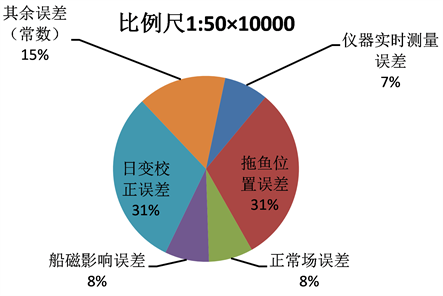(a)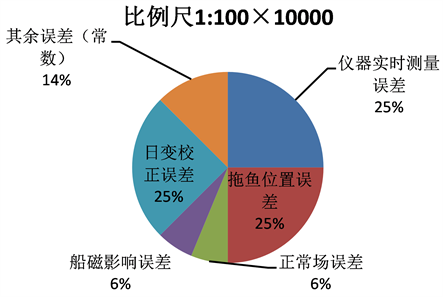(b)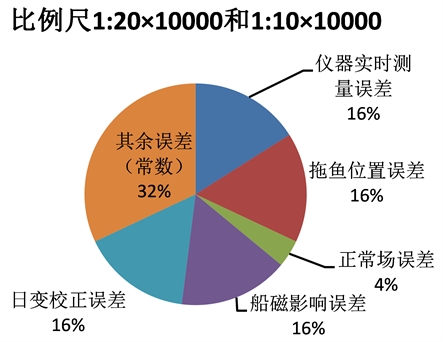(c)

Figure 1. Ti takes the maximum value of the total error pie chart with different error ranges under different scales. (a) When Ti has a maximum scale of 1:100 × 104, the total error pie chart with different error ranges; (b) When Ti has a maximum scale of 1:50 × 104, the total error pie chart with different error ranges; (c) When Ti has a maximum scale of 1:20 × 104 and 1:10 × 104, the total error pie chart with different error ranges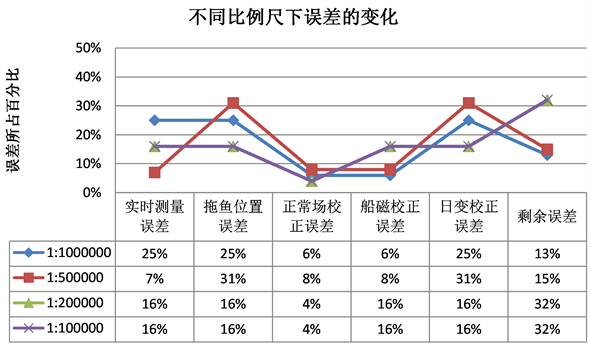Figure 2. The change of error at different scales when Ti takes the maximum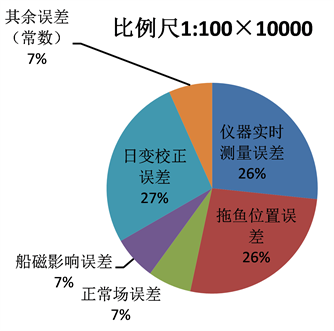(a)(b)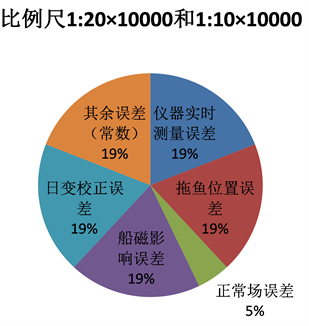(c)

Figure 3. Ti takes the median value of the total error pie chart with different error ranges under different scales. (a) When Ti takes a median scale of 1:100 × 104, the total error pie chart with different error ranges; (b) When Ti takes a median scale of 1:50 × 104, the total error pie chart with different error ranges; (c) When Ti takes a median scale of 1:20 × 104 and 1:10 × 104, the total error pie chart with different error ranges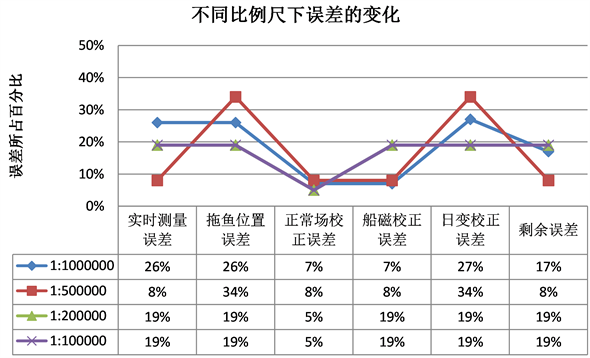Figure 4. Change of error at different scales when Ti takes the median

2) 随着比例尺的增大，实时测量误差即仪器误差的影响所占比例先缩小后变大，在实际海洋磁力测量过程中，要根据比例尺的实际情况做出相应的调整；拖鱼位置误差和日变校正误差基本一致，随着比例尺的增大，先增大后减小，并且在 $1:50×{10}^{4}$ 时所占比例很大，建议在 $1:50×{10}^{4}$ 度时，可以适当提高拖鱼位置和日变校正的准确度减小两者的误差，从而对减小总误差有明显的作用；正常场校正误差所占比例变化不大且较小；船磁校正误差随着比例尺精度增大而增大，在实际测量中随着精度要求的增大，要削弱船磁校正误差的影响；数据整理、处理过程产生的误差和海洋磁力测量实施过程中的误差在小比例尺测量时，影响程度不大，但是在大比例尺，海洋磁力测量精确度高的作业中，数据整理、处理过程产生的误差和海洋磁力测量实施过程中的误差成为主要误差。

3) 海洋磁力测量的误差是多项因素的综合误差，因此，对于其对海洋磁力测量的影响应综合考虑。本文建立了海洋磁力测量的数据误差处理模型，对影响海洋磁力测量的各项误差进行了分析，得出各误差在不同比例尺的海洋磁力测量中误差的变化规律，给出了海洋磁力测量误差分配方案，在确定海洋磁力测量的比例尺精确度要求后，一方面应该在测量实施时对各种误差的影响进行控制，另一方面应对海洋磁力测量的影响综合考虑，根据各项误差分配方案采取措施着重消除突出误差部分。

  梁开龙. 海洋重力测量与磁力测量[M]. 北京: 测绘出版社, 1996.  边刚, 刘雁春, 暴景阳, 等. 海洋磁力测量误差源分析[C]//中国测绘学会. 第十五届海洋测绘综合性学术研讨会论文集, 2003: 98-100.  海洋调查规范•海洋地质地球物理调查. GB/T13909.1992.  裴彦良, 梁瑞才, 刘晨光, 等. 海洋磁力仪的原理与技术指标对比分析[J]. 海洋科学, 2005, 29(12): 4-8.  王亶文. 国际地磁参考场在中国大陆地区的误差分析[J]. 地球物理学报, 2003, 46(2): 171-174.  Riddihough, R.P. (1971) Diurnal Corrections to Magnetic Surveys—An Assessment of Errors. Geophysical Prospecting, 19, 551-567. https://doi.org/10.1111/j.1365-2478.1971.tb00900.x  邵关. 海底地磁日变观测安全布放技术[J]. 海洋测绘, 2013, 33(1): 69-71.  高金耀, 刘强, 翟国君, 等. 与海洋地磁日变改正有关的长期变化和磁扰的处理[J]. 海洋学报, 2009, 31(4): 88-92.  任来平, 张襄安, 刘国斌. 海洋磁力测量系统误差来源分析[J]. 海洋测绘, 2004, 24(5): 5-8.  夏伟, 边刚, 金绍华, 等. 海面与海底地磁日变化差异及其对海洋磁力测量的影响[J]. 海洋测绘, 2015, 35(1): 7-10.  边刚, 卞光浪, 刘雁春, 等. 海洋磁力测量中地磁日变信号的经典功率谱估计[C]//中国测绘学会. 第十八届海洋测绘综合性学术研讨会论文集, 2006: 55-58.  边刚, 刘雁春, 翟国君,等. 海洋磁力测量拖鱼位置概算[J]. 测绘通报, 2004(8): 9-11.  韩范畴, 任来平. 应用傅立叶级数计算船尾磁场[J]. 海洋测绘, 2003, 23(3): 5-10.